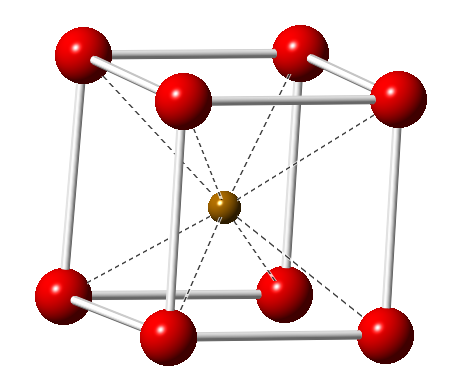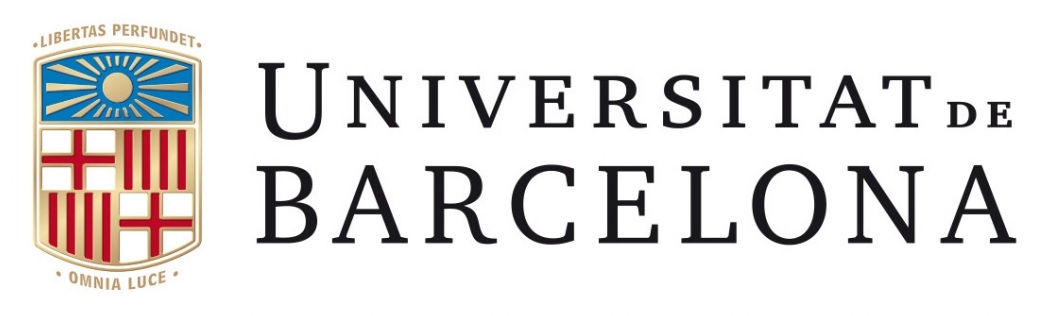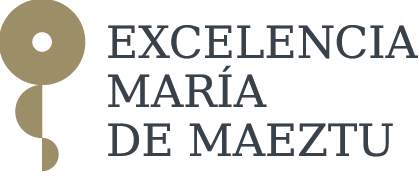# cosymlib¶

Cosymlib is a python library for computing continuous symmetry & shape measures (CSMs & CShMs). Although its main aim is to provide simple and ready-to-use tools for the analysis of the symmetry & shape of molecules, many of the procedures contained in cosymlib can be easily applied to any finite geometrical object defined by a set of vertices or a by mass distribution function.

The basic tasks included in the current version of cosymlib are:

1. Molecular structure analysis

• Calculation of Continuous Shape Measures (CShMs)
• Calculation of Continuous Symmetry Measures (CSMs)
• Calculation of Continuous Chirality Measures (CCM)
2. Electronic structure analysis

• Pseudosymmetry analysis of molecular orbitals & wavefunctions
• Continuous Symmetry & Chirality Measures for the molecular electron density

Cosymlib is being developed by the Electronic Structure & Symmetry (ESS) group at the Department de Ciència de Material i Química Física and the Institut de Química Teòrica i Computacional (IQTCUB). University of Barcelona.